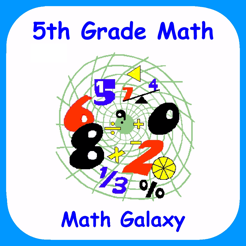## Insight scoop about 5th Grade Math Common Core by Math Galaxy### Description:

In fifth grade math the emphasis is on fractions, decimals and volume: (1) developing fluency with addition and subtraction of fractions and multiplication and division of fractions and whole numbers, (2) decimal operations to hundredths and whole number division with 2-digit divisors, and (3) volume.

Math Galaxys Fifth Grade Common Core Math covers all of the Common Core math standards for fifth grade as listed below.
The concepts are presented in bite-size pieces with an unlimited supply of practice problems, with numbers chosen randomly.
Illustrations are used throughout to reinforce the concepts.
By solving problems students earn robots to use in a maze game, plus there are several other games involving math practice.
Certificates with scores can be emailed to be printed.

NUMBERS AND OPERATIONS IN BASE 10 (5.NBT)

Place Value (5.NBT.1, 5.NBT.3a)
Place Names
Expanded Notation

Regrouping (5.NBT.1)
(Whole #s & Decimals)

Comparing Decimals (5.NBT.3b)

Rounding Decimals (5.NBT.4)

Multiplying & Dividing by 10s (5.NBT.2)

Multiplying Whole Numbers (5.NBT.5)

Dividing Whole Numbers (5.NBT.6)
(2-3 Digit Divisors, Traditional k& Alternative)

Decimal Operations (5.NBT.7)

FRACTIONS (5.NF)

Multiplying Fractions (5.NF.4)
Understanding Fractions as Division (5.NF.3)
Dividing Unit Fractions (5.NF.7)
Dividing Simple Fractions (5.NF.7)
Fraction Word Problems (5.NF.2, 5.NF.6, 5.NF.7c)

MEASUREMENT (5.MD)

Length (5.MD.1)
Area (5.MD.1)
Mass (5.MD.1)

Volume
Converting Units (5.MD.1)
Cubic Units 1 (5.MD.3, 5.MD.4)
Cubic Units 2 (5.MD.5c)
Missing Side (5.MD.5a, 5b)

GEOMETRY (5.G)

Graphing Coordinates (5.G1, 5.G2)
Lines, Angles, & Polygons (5.G.3, 5.G.4)

ALGEBRAIC OPERATIONS (5.OA)

Algebraic Operations (5.OA.1, 5.OA.2)
Algebraic Patterns (5.OA.3)

GAMES

The Money Trail
Bridge the Swamp (adding, subtracting, multiplying, & dividing fractions and decimals)
Riddles (Length, Area, Volume, & Time - in Measurement section)
Labyrinth (a maze game - robots are earned in exercises.)

Report an Error